# NCERT Solutions for Class 10 Maths Chapter 3 Pair of Linear Equations in Two Variables Ex 3.3

Get Free NCERT Solutions for Class 10 Maths Chapter 3 Ex 3.3 PDF. Pair of Linear Equations in Two Variables Class 10 Maths NCERT Solutions are extremely helpful while doing your homework. Exercise 3.3 Class 10 Maths NCERT Solutions were prepared by Experienced ncert-books.in Teachers. Detailed answers of all the questions in Chapter 3 Maths Class 10 Pair of Linear Equations in Two Variables Exercise 3.3 provided in NCERT TextBook.

Topics and Sub Topics in Class 10 Maths Chapter 3 Pair of Linear Equations in Two Variables:

 Section Name Topic Name 3 Pair of Linear Equations in Two Variables 3.1 Introduction 3.2 Pair Of Linear Equations In Two Variables 3.3 Graphical Method Of Solution Of A Pair Of Linear Equations 3.4 Algebraic Methods Of Solving A Pair Of Linear Equations 3.4.1 Substitution Method 3.4.2 Elimination Method 3.4.3 Cross-Multiplication Method 3.5 Equations Reducible To A Pair Of Linear Equations In Two Variables 3.6 Summary

You can also download the free PDF of  Ex 3.3 Class 10 Pair of Linear Equations in Two Variables NCERT Solutions or save the solution images and take the print out to keep it handy for your exam preparation.

 Board CBSE Textbook NCERT Class Class 10 Subject Maths Chapter Chapter 3 Chapter Name Pair of Linear Equations in Two Variables Exercise Ex 3.3 Number of Questions Solved 3 Category NCERT Solutions

## NCERT Solutions for Class 10 Maths Chapter 3 Pair of Linear Equations in Two Variables Ex 3.3

NCERT Solutions for Class 10 Maths Chapter 3 Pair of Linear Equations in Two Variables Ex 3.3 are part of NCERT Solutions for Class 10 Maths. Here we have given NCERT Solutions for Class 10 Maths Chapter 3 Pair of Linear Equations in Two Variables Exercise 3.3

Question 1.
Solve the following pairs of linear equations by the substitution method:Solution: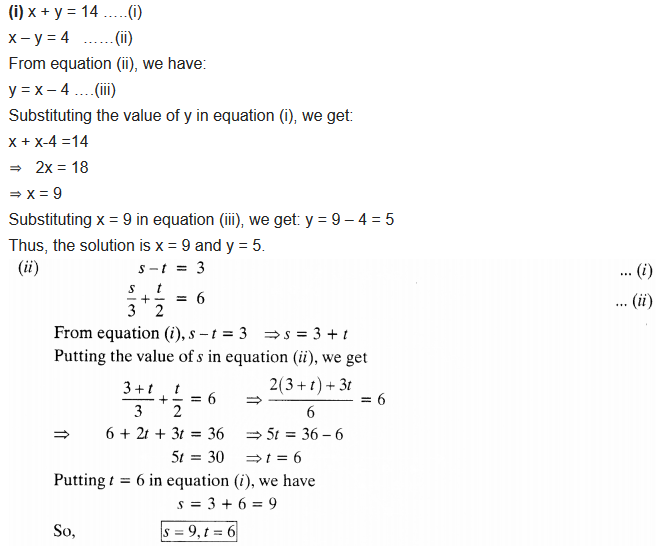Question 2.
Solve 2x + 3y = 11 and 2x – 4y = -24 and hence find the value of’m’ for which y = mx +3.
Solution: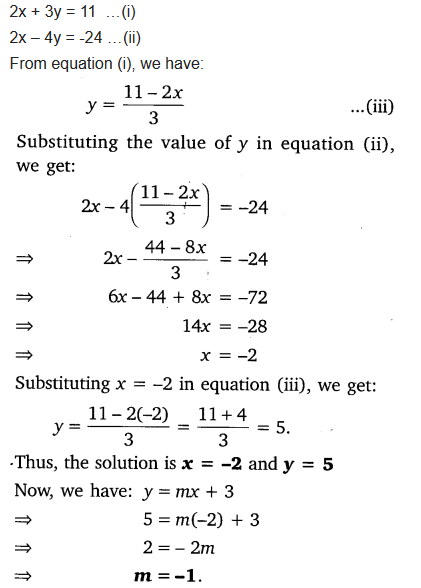Question 3.
Form the pair of linear equations for the following problems and find their solution by substitution method:
(i) The difference between two numbers is 26 and one number is three times the other. Find them.
(ii) The larger of two supplementary angles exceeds the smaller by 18 degrees. Find them.
(iii) The coach of a cricket team buys 7 bats and 6 balls for ₹3800. Later, she buys 3 bats and 5 balls for ₹1750. Find the cost of each bat and each ball.
(iv) The taxi charges in a city consist of a fixed charge together with the charge for the distance covered. For a distance of 10 km, the charge paid is ₹105 and for a journey of 15 km, the charge paid is ₹155. What are the fixed charges and the charges per km? How much does a person have to pay for travelling a distance of 25 km?
(v) A fraction becomes$\frac { 9 }{ 11 }$, if 2 is added to both the numerator and the denominator. If 3 is added to both the numerator and the denominator, it becomes$\frac { 5 }{ 6 }$. Find the fraction.
(vi) Five years hence, the age of Jacob will be three times that of his son. Five year ago, Jacob’s age was seven times that of his son. What are their present ages?
Solution: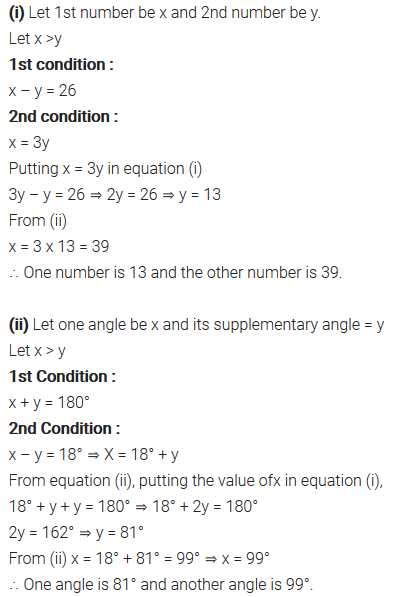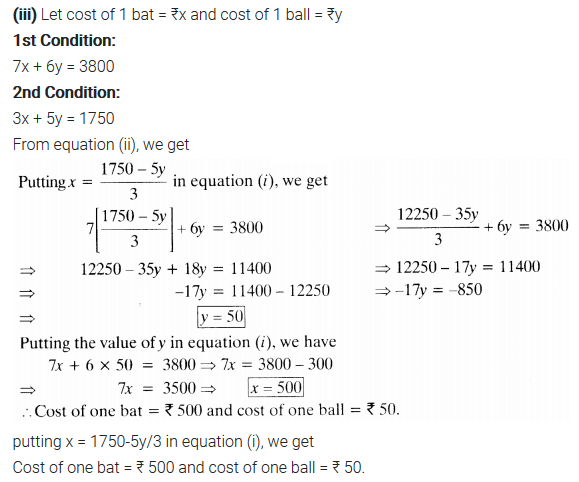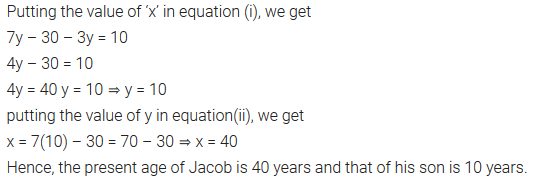+